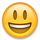# Removing rows from a table based on a column value

Hi there, I'm attempting to remove rows from a table based on values out of column 0 of the table. For ex. if column 0 == 3, then remove the row. Is there any way to do this, maybe using a filter? or a function within rows()?

• One way of attempting to remove rows is to use `filter()` and `rows().remove()`. Try the below example and update as needed:

``````                              var filteredData = table
.rows()
.indexes()
.filter( function ( value, index ) {
return table.row(value).data() == 3;
} );
table.rows( filteredData )
.remove()
.draw();
``````

This gets the row indexes where column 0 == 3 and stores them in `filteredData`. `filteredData` is then used as the row selector and those rows are removed and the table redrawn. You may need to change the column number in line 5 to the name of the column depending on your config.

Kevin

• Good thinking Kevin!

Another option is to use the `row-selector` option as a function:

``````table
.rows( function ( idx, data, node ) {
return data === 3;
} )
.remove()
.draw();
``````

Its basically the same thing, just using a little shortcut.

Allan

• Hey guys! Just wanna point out something that had me deleting the opposite rows that I needed:

In @kthorngren 's answer it's supposed to be

``````.filter( function ( index, value) {
``````

I mean, it's more descriptive that way. I was using the "index" to get at my rows and what I was actually using was the value.
Still, this is what solved my day-long problem! Thank you so muchThis discussion has been closed.